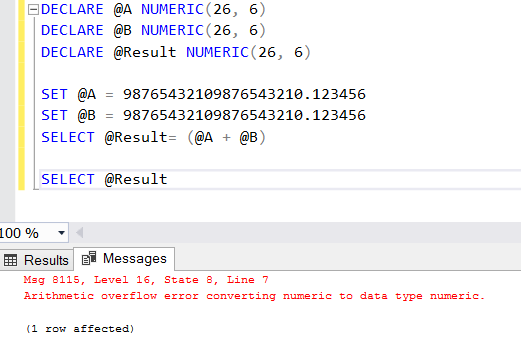Friday , July 1 2022

# Arithmetic overflow error converting numeric to data type numeric.

### ERROR MESAGGE:

“Arithmetic overflow error converting numeric to data type numeric.”

### EXPLANATION:

You may receive this error message when you are using data types such as DECIMAL, NUMERIC.

The reason for the error is that the data type of the variable to be set is not configured correctly.

Let me explain with an example. In the example I define three variables.

• The first variable “A” is any number
• The second variable “B” is any number
• The third variable is “Result”; We will set the sum of “A” and “B” variables to this variable.

Below you can see the script that performs these operations.

When you run this script, you will see an “Arithmetic overflow error converting numeric to data type numeric.” error as follows.In order to better explain this problem, let me briefly mention what “NUMERIC (26, 6)” means for friends who do not know.

The number 26 in the parenthesis is the total number of digits on the left and right of the comma. A maximum of 38 numbers can be used for this purpose.

The number 6 in the parenthesis and to the right of the comma gives the digit length in the decimal part of the number in the Numeric data type.

If you look at our example, there are exactly 20 digits to the left of the dot and 6 to the right. 26 in total. If this digits becomes 27, you will encounter this error.

### SOLUTION:

I’ll reach the solution by continuing from the above example.

The reason we encounter this error is that our result is a number consisting of 27 digits. That is, the sum of the variables “A” and “B” includes a number of 27 digits. If we define the “Result” variable in our example with the DECLARE command and define it as NUMERIC (27,6), then the result will be retrieved without error.##### Author: Ekrem Önsoy

The original article was written in Turkish by Ekrem Önsoy and translated to English by dbtut with the consent of the author. The copyright of the article belongs to the author. The author shall not be liable in any way for any defect caused by translation.